### 7 Matchstick Equation Puzzles

In matchstick puzzles, you are presented with an incorrect equation made using matchsticks, and you must move 1 or more matchsticks to turn it into a valid equation.

You must use all of the matches, and you are not allowed to make an inequality symbol such as ≠, ≥, >, <, or ≤.

Try these 7 fun matchstick equation puzzles!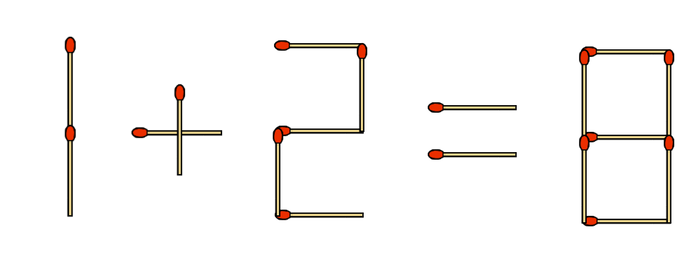Click for Solution to 1 + 2 = 8

7 + 2 = 9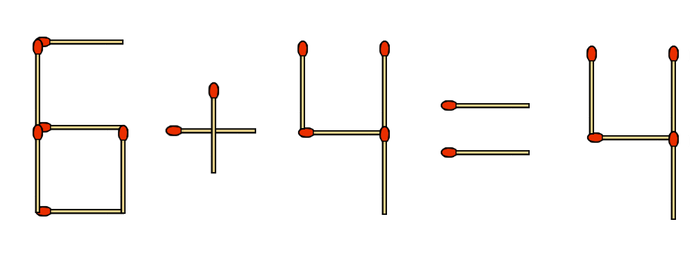Click for Solution to 6 + 4 = 4

8 – 4 = 4Click for Solution to 4 + 4 = 7

11 – 4 = 7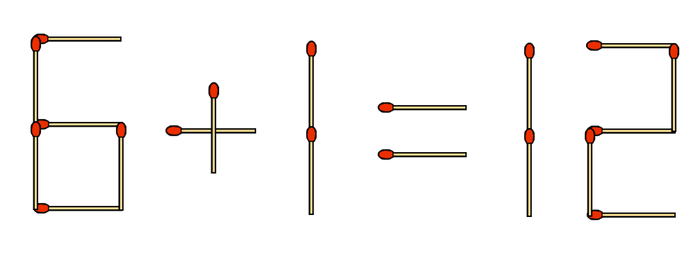Click for Solution to 6 + 1 = 12

5 + 7 = 12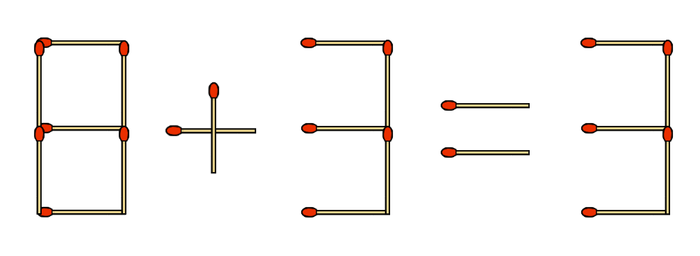Click for Solution to 8 + 3 = 3

6 + 3 = 9Click for Solution to 5 + 9 = 9

5 + 3 = 8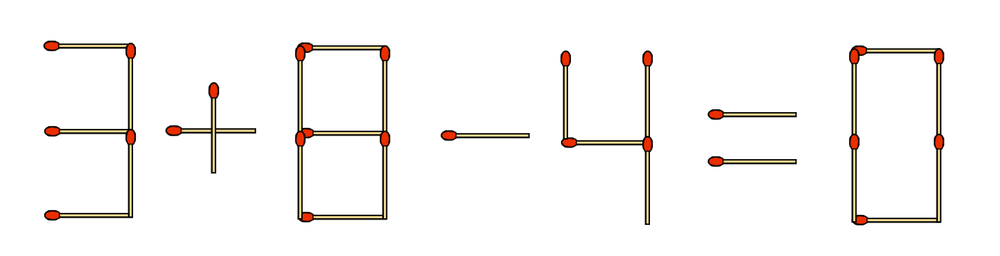Bonus: find 3 different solutions!

Click for Solution to 3 + 8 – 4 = 0

3 + 9 – 4 = 8

3 + 6 – 9 = 0

3 + 8 – 11 = 0